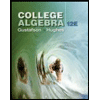# Use this fact and  find the acute angles between the lines in Exercises 47–51. 47. 3x + y = 5, 2x - y = 4 48. y = sqrt(3)x - 1, y =-sqrt(3)x + 2 49. sqrt(3)x - y =-2, x - sqrt(3)y = 1  50. 3x - 4y = 3, x - y = 7 51. 12x + 5y = 1, 2x - 2y = 3

Question

Use this fact and  find the acute angles between the lines in Exercises 47–51. 47. 3x + y = 5, 2x - y = 4

48. y = sqrt(3)x - 1, y =-sqrt(3)x + 2

49. sqrt(3)x - y =-2, x - sqrt(3)y = 1

50. 3x - 4y = 3, x - y = 7

51. 12x + 5y = 1, 2x - 2y = 3

Expert Solution

### Want to see the full answer?

Check out a sample Q&A hereStudents who’ve seen this question also like:College Algebra (MindTap Course List)
12th Edition
ISBN: 9781305652231
Author: R. David Gustafson, Jeff Hughes
Publisher: Cengage Learning